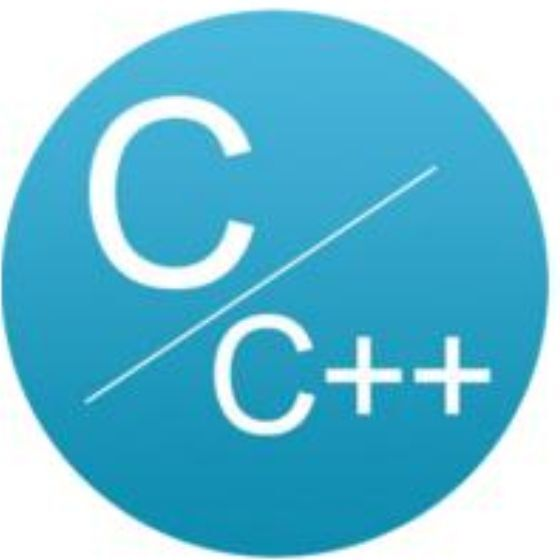# 简述 C 语言宏定义的使用C语言与CPP编程

## 1 概述

1. 简单宏定义

#define 标识符 字符串

// 不带参数的宏定义#define MAX 10

• 程序会更易读。一个认真选择的名字可以帮助读者理解常量的意义；

• 程序会更易于修改。我们仅需要改变一个宏定义，就可以改变整个程序中出现的所有该常量的值；

• 可以帮助避免前后不一致或键盘输入错误；

• 控制条件编译；

• 可以对C语法做小的修改；

1. 带参数的宏

#define <宏名>(<参数列表>) <宏体>

#define COUNT(M) M*Mint x=5;print(COUNT(x+1));print(COUNT(++X));//结果输出：11   和42 而不是函数的输出36

• 预编译器只是进行简单的文本替换，COUNT(x+1)被替换成COUNT(x+1x+1)，5+15+1=11，而不是36

• CUNT(++x)被替换成++x*++x即为6*7=42，而不是想要的6*6=36，连续前置自加加两次

• 用括号将整个替换文本及每个参数用括号括起来print(COUNT((x+1));

• 即便是加上括号也不能解决第二种情况，所以解决办法是尽量不使用++，-等符号；

#define foo(x) bar(x); baz(x)

if (!feral)    foo(wolf);

﻿

if (!feral)    bar(wolf);baz(wolf);

﻿

==baz(wolf);==,不在判断条件中，显而易见，这是错误。如果用大括号将其包起来依然会有问题，例如

#define foo(x)  { bar(x); baz(x); }if (!feral)    foo(wolf);else    bin(wolf);

if (!feral) {    bar(wolf);    baz(wolf);}>>++;++<<else    bin(wolf);

==else==将不会被执行

#define foo(x)  do{ bar(x); baz(x); }while(0)if (!feral)    foo(wolf);else    bin(wolf);

#define foo(x)  do{ bar(x); baz(x); }while(0)if (!feral)    do{ bar(x); baz(x); }while(0);else    bin(wolf);

1. #运算符

#的作用就是将#后边的宏参数进行字符串的操作，也就是将#后边的参数两边加上一对双引号使其成为字符串。例如a是一个宏的形参，则替换文本中的#a被系统转化为"a",这个转换过程即为字符串化。

#define TEST(param) #paramchar *pStr=TEST(123);printf("pSrt=%s\n",pStr);//输出结果为字符  ”123“
1. ##运算符

##运算符也可以用在替换文本中，它的作用起到粘合的作用，即将两个宏参数连接为一个数

#define TEST(param1,param2) (param1##param2)int num =TEST(13,59);printf("num=%d\n",num);//输出结果为：num=1359
1. VA_ARGS

 # define PR(...) printf(_VA_ARGS_) PR("hello world\n"); 输出结果：hello world

## 2 一些建议

• 虽然宏定义很灵活，并且通过彼此结合可以产生许多变形用法，但是C++/C程序员不要定义很复杂的宏，宏定义应该简单而清晰。

• 宏名采用大写字符组成的单词或其缩写序列，并在各单词之间使用“_”分隔。

• 如果需要公布某个宏，那么该宏定义应当放置在头文件中，否则放置在实现文件（.cpp）的顶部。

• 不要使用宏来定义新类型名，应该使用typedef，否则容易造成错误。

• 给宏添加注释时请使用块注释（/* */），而不要使用行注释。因为有些编译器可能会把宏后面的行注释理解为宏体的一部分。

• 尽量使用const取代宏来定义符号常量。

• 对于较长的使用频率较高的重复代码片段，建议使用函数或模板而不要使用带参数的宏定义；而对于较短的重复代码片段，可以使用带参数的宏定义，这不仅是出于类型安全的考虑，而且也是优化与折衷的体现。

• 尽量避免在局部范围内（如函数内、类型定义内等）定义宏，除非它只在该局部范围内使用，否则会损害程序的清晰性。

## 3 宏的常见用法

• 防止一个头文件被重复包含

#ifndef COMDEF_H#define COMDEF_H//头文件内容#endif

#define  MEM_B(x) (*((byte *)(x)))#define  MEM_W(x) (*((word *)(x)))

#define  MAX(x,y) (((x)>(y)) ? (x) : (y))#define  MIN(x,y) (((x) < (y)) ? (x) : (y))

#define FPOS(type,field) ((dword)&((type *)0)->field)

#define FSIZ(type,field) sizeof(((type *)0)->field)

#define FLIPW(ray) ((((word)(ray)) * 256) + (ray))

#define WORD_LO(xxx)  ((byte) ((word)(xxx) & 255))#define WORD_HI(xxx)  ((byte) ((word)(xxx) >> 8))

#define UPCASE(c) (((c)>='a' && (c) <= 'z') ? ((c) – 0×20) : (c))

#define  DECCHK(c) ((c)>='0' && (c)<='9')

#define HEXCHK(c) (((c) >= '0' && (c)<='9') ((c)>='A' && (c)<= 'F') \((c)>='a' && (c)<='f'))

#define INC_SAT(val) (val=((val)+1>(val)) ? (val)+1 : (val))

#define ARR_SIZE(a)  (sizeof((a))/sizeof((a)))

## 参考资料

1. http://www.360doc.com/content/13/0125/13/10906019_262310086.shtml

2. 高质量程序设计指南C++/C语言第3版

3. https://www.cnblogs.com/southcyy/p/10155049.html

﻿﻿﻿

﻿## 评论